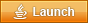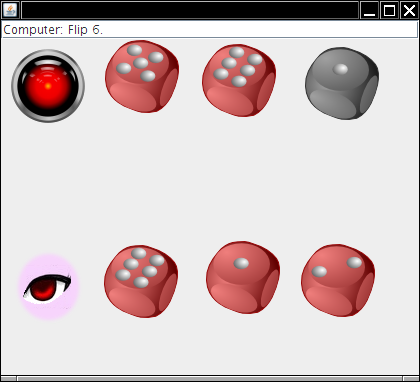# Flip with Prolog

In the game of Nim, two players alternately remove marbles from a pile of marbles. Here is a particularly simple variation. In each turn, a player must remove at least one marble, and at most half the marbles. The following predicate tests whether it a move from X marbles to Y marbles is a legal move:

`move(X,Y) :- X > Y, X =< 2 * Y.`

A position consisting of two marbles is a winning position:

`win(X) :- X=:=2.`

This game has a well-known winning strategy that you should figure out. (Hint: Look at piles of size 2k - 1...)

According to the textbook, we should be able to test whether any position is a winning position by adding the following clause:

`win(X) :- move(X,Y), not win(Y).`

However, that doesn't work.

```?- win(4).
ERROR: Arguments are not sufficiently instantiated```

There are two ways for fixing this. You can describe the state with a list, not an integer: [o,o,o,o,o] would denote five marbles.

```movel(X,Y) :- append(_,Y,X), length(X,LX), length(Y,LY), LX > LY, LX =< 2 * LY.
winl(X) :- length(X,LX), LX=:=2.
winl(X) :- movel(X,Y), not(winl(Y)).```

Or you can use the technique that we used for solving for factorials in the lab.

```moven(X,Y) :- numlist(1,X,S), member(Y,S), X > Y, X =< 2 * Y.
winn(X) :- X=:=2.
winn(X) :- moven(X,Y), not(winn(Y)).```

All this is just by way of introduction. The game that we want to study is Flip. To understand the rules, it is helpful to practice playing with a (very stupid) opponent:Program doesn't start? Install the latest version of Java:Once you understand the rules, spend some time playing Flip with your buddy so you get a feel for strategy.

Just like with Nim, you need to represent the game state: the positions of all the dice in the game. Note the stalemate rule. You need to keep track of the dice that are not flippable.

There is a particular subtlety in Flip. We want to carefully separate the decisions of each player. Suppose your opponent "plays" you. It is your opponent's decision which of your dice to put into the middle. But it is your decision which dice to take from the middle. Therefore, we will consider your opponent's turn to end immediately after the "play". Your turn will begin by taking dice from the middle and end with your flip or "play".

In summary, the Flip game state consists of

• the flippable and unflippable dice of each player
• the dice in the middle
• the die in the middle that was just "played" (if any)

In Prolog, a simple representation would be a list of lists, e.g. [, , [5, 2], [], [4, 2], 2] to denote that player 1 has one flippable and one unflippable die, player 2 has two dice, both flippable, and dice with values 4 and 2 are in the middle, with 2 just played.

However, that representation may not be so good. Consider this `flip` predicate, where

`flip(BF, BN, AF, AN)`

is true if your flippable/non-flippable die lists BF/BN are transformed into AF/AN by a flip. That's not too hard. One of the elements of BF must have made it into AN after being flipped.

```flip(1,6).
flip(2,5).
flip(3,4).
flip(4,3).
flip(5,2).
flip(6,1).
flip(BF, BN, AF, AN) :- select(X, BF, AF), flip(X, Y), append(BN, [Y], AN). ```

Now try

```flip([3,3],[],X,Y).

X = 
Y =  ;

X = 
Y =  ;

No```

You get two identical answers. For efficiency of searching, you do not want to produce duplicate moves. You should use a more sophisticated representation (for example, sorted lists or frequency tables). Design custom predicates for making selections and unions in your data structure.

Produce the following predicates:

`move(X, Y)`

Enumerates all legal moves with starting position X.

`win(X)`

True if X is a winning position.

`next(X, Y)`

Enumerates the optimal move(s) from position X.

Finally, list all winning positions with two dice and count all winning positions with 3 dice. Determine the probability that a random starting position is a winning position for 2 and 3 dice.

Note: Because of the design aspect, you will need to put in sustained trial and error work for several weeks, and you will need to be able to live with, and learn from, failure. You will need to turn in intermediate progress reports every Monday, and you will need to be prepared to discuss your experiments and observations in class.